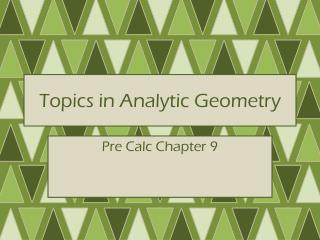DownloadDownload PresentationTopics in Analytic Geometry

Topics in Analytic Geometry

Download PresentationTopics in Analytic Geometry

- - - - - - - - - - - - - - - - - - - - - - - - - - - E N D - - - - - - - - - - - - - - - - - - - - - - - - - - -
Presentation Transcript

1. Topics in Analytic Geometry Pre Calc Chapter 9

2. Parabolas—9.1

3. Parabolas • Vertex • The lowest or highest point of the graph (based on which way it opens) • Axis of symmetry

4. Geometric Definition • A parabola is the set of points in the plane equidistant from a fixed point F (the focus) and a fixed line l called the directrix

5. Parabolas

6. Analytic Geometry • Concerned with shapes, not necessarily functionality • Parabolas can open up • Parabolas can open down • Inverse

7. Parabola with Vertical Axis The Graph of the equation:is a parabola with the following: Vertex Focus Directrix Parabola opens up if or down if

8. Parabola with Horizontal Axis The Graph of the equation:is a parabola with the following: Vertex Focus Directrix Parabola opens right if or left if

9. Focal Diameter • Distance across the parabola along the line parallel to the directrix

10. Examples

11. Examples

12. Examples

13. p.730#1-10,25-27,37-40

14. Ellipses—9.2

15. Geometric Definition • An ellipse is the set of all points in the plane the sum of whose distances from two fixed points and is a constant. • These points are the foci

16. Ellipse Equation Vertices Major Axis Horizontal, length 2a Vertical, 2a Minor Axis Vertical, length 2b Horizontal, 2b Foci

17. Ellipses

18. Ellipses

19. Ellipses

20. Eccentricity • For the ellipse or the eccentricity, e, is the numberwhere and the eccentricity of every ellipse satisfies

21. Ellipses • Find the equation of the ellipse with foci and eccentricity

22. p.741#1-8,19-22,29-31

23. Hyperbolas—9.3

24. Geometric Definition • A hyperbola is the set of all points in the plane, the difference of whose distances from two fixed points and is a constant. • These are the foci of the hyperbola

25. Hyperbolas Equations Vertices Transverse Axis Horizontal, length 2a Asymptotes Foci

26. Hyperbolas Equations Vertices Transverse Axis Vertical, length 2b Asymptotes Foci

27. Sketching Hyperbolas • Sketch the Central Box • Sketch the asymptotes • Plot the vertices • Sketch the hyperbola • Smile 

28. Hyperbolas

29. Hyperbolas

30. Hyperbolas • Find the equation of the hyperbola with vertices and foci

31. p.751#1-8,17-19

32. Shifted Conics—9.4

33. Shifting Conics

34. Shifted Conics

35. Shifted Conics

36. Shifted Conics

37. General Equation of a Conic • The graph of the equationWhere A and C are not both 0, is a conic or degenerate conic where the graph is: • A parabola if A or C is 0 • An ellipse if A and C have the same sign • Circle if A = C • A hyperbola is A and C have opposite signs

38. Conics

39. Degenerate Conic • Conic which simplifies to only 2 lines

40. p.760#1,2,5,6,9,10, 19-23 (10 pt)

41. Rotation of Axes—9.5

42. Rotation of Axes • Recall… • Now…

43. Rotation of Axes Formula • Suppose the x- and y-axes in a coordinate plane are rotated through the acute angle to produce the X- and Y-axes. Then the coordinates (x,y) and (X,Y) of a point in the xy- and XY-panes are related as follows: x=Xcosφ-Ysinφ X=xcosφ+ysinφ y=Xsinφ+Ycosφ Y=-xsinφ+ycosφ

44. Rotation of Axes • If the coordinates are rotated 30 degrees, find the XY-coordinates of the point with xy-coordinates (2, -4)

45. Rotation of Axes • Rotate the coordinate axes through 45 degrees to show that the graph of the equation xy = 2 is a hyperbola

46. Identifying Conics by the Discriminant

47. Polar Coordinates—9.6

48. Polar Coordinates • Uses distances and directions to specify locations on the plane • Origin (Pole) • Polar Axis • Polar Coordinates